Test: Binomial Theorem - 6

# Test: Binomial Theorem - 6

Test Description

## 20 Questions MCQ Test Topic-wise Tests & Solved Examples for IIT JAM Mathematics | Test: Binomial Theorem - 6

Test: Binomial Theorem - 6 for Mathematics 2023 is part of Topic-wise Tests & Solved Examples for IIT JAM Mathematics preparation. The Test: Binomial Theorem - 6 questions and answers have been prepared according to the Mathematics exam syllabus.The Test: Binomial Theorem - 6 MCQs are made for Mathematics 2023 Exam. Find important definitions, questions, notes, meanings, examples, exercises, MCQs and online tests for Test: Binomial Theorem - 6 below.
Solutions of Test: Binomial Theorem - 6 questions in English are available as part of our Topic-wise Tests & Solved Examples for IIT JAM Mathematics for Mathematics & Test: Binomial Theorem - 6 solutions in Hindi for Topic-wise Tests & Solved Examples for IIT JAM Mathematics course. Download more important topics, notes, lectures and mock test series for Mathematics Exam by signing up for free. Attempt Test: Binomial Theorem - 6 | 20 questions in 60 minutes | Mock test for Mathematics preparation | Free important questions MCQ to study Topic-wise Tests & Solved Examples for IIT JAM Mathematics for Mathematics Exam | Download free PDF with solutions
 1 Crore+ students have signed up on EduRev. Have you?
Test: Binomial Theorem - 6 - Question 1

### {an} and {bn} be two sequences given by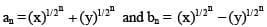for all n∈N, then a1 a2 a3 … an is equal to

Detailed Solution for Test: Binomial Theorem - 6 - Question 1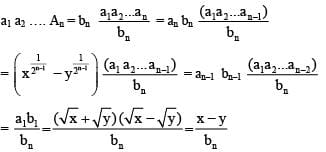Test: Binomial Theorem - 6 - Question 2

###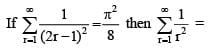Test: Binomial Theorem - 6 - Question 3

### If a, b, c ∈R+ form a A.P., then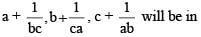Detailed Solution for Test: Binomial Theorem - 6 - Question 3

Since a, b, c are in A.P., therefore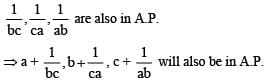Test: Binomial Theorem - 6 - Question 4

If Zr, r = 1, 2, ...,100 are the roots of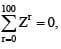then the value of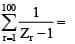Detailed Solution for Test: Binomial Theorem - 6 - Question 4

zr are the roots of z101 − 1 =0 , except 1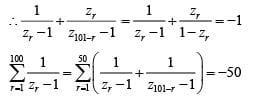Test: Binomial Theorem - 6 - Question 5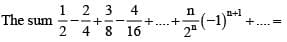Detailed Solution for Test: Binomial Theorem - 6 - Question 5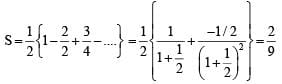Test: Binomial Theorem - 6 - Question 6

The sum of coefficients of (1 + x − 3x2)2134 is

Detailed Solution for Test: Binomial Theorem - 6 - Question 6

For the sum of coefficient, put x = 1, to obtain the sum is (1 + 1 ‐ 3)2134 = 1

Test: Binomial Theorem - 6 - Question 7

The sum rCr + r+1Cr + r+2Cr + .... + nCr (n > r) equals

Detailed Solution for Test: Binomial Theorem - 6 - Question 7

C(n, r) + c(n ‐1, r) + C(n ‐ 2, r) + . . . + C(r, r)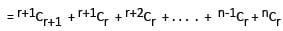= n+1Cr+1 (applying same rule again and again)
(∵ nCr + nCr‐1 = n+1Cr)

Test: Binomial Theorem - 6 - Question 8

The expansion [x2 + (x6 - 1)1/2]5 + [x2 - (x6 - 1)1/2]5 is a polynomial of degree

Detailed Solution for Test: Binomial Theorem - 6 - Question 8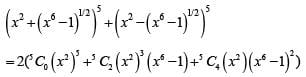Here last term is of 14 degree.

Test: Binomial Theorem - 6 - Question 9

The term independent of x in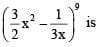Detailed Solution for Test: Binomial Theorem - 6 - Question 9

The general term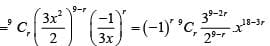The term independent of x, (or the constant term) corresponds to x18−3r being xor 18 − 3r= 0 ⇒ r = 6 .

Test: Binomial Theorem - 6 - Question 10

The value of the greatest term in the expansion of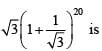Detailed Solution for Test: Binomial Theorem - 6 - Question 10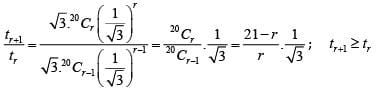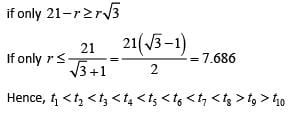Hence, t8 is the greatest term and its value is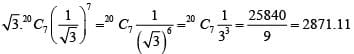Test: Binomial Theorem - 6 - Question 11

9n +1 − 8n − 9 is divisible by

Detailed Solution for Test: Binomial Theorem - 6 - Question 11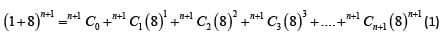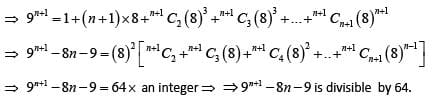Test: Binomial Theorem - 6 - Question 12

The first integral term in the expansion of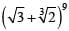is its

Detailed Solution for Test: Binomial Theorem - 6 - Question 12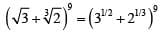∴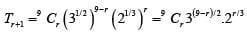For first integral term for r = 3;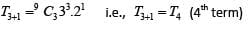Test: Binomial Theorem - 6 - Question 13

The number of irrational terms in the expansion of (21/5 + 31/10)55 is

Detailed Solution for Test: Binomial Theorem - 6 - Question 13

(21/5 + 31/10)55
Total terms = 55 + 1 = 56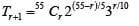Here r = 0, 10, 20, 30, 40, 50
Number of rational terms = 6;
Number of irrational terms = 56 ‐ 6 = 50

Test: Binomial Theorem - 6 - Question 14

The number of terms in the expansion of (2x + 3y− 4z)n is

Detailed Solution for Test: Binomial Theorem - 6 - Question 14

We have, (2x + 3y − 4z)n = {2x + (3y − 4z)}n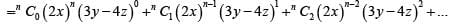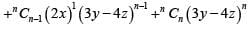Clearly, the first term in the above expansion gives one term, second term gives two terms, third term gives three terms and so on.
So, Total number of term = 1 +2+3+...+n+(n+1)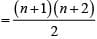Test: Binomial Theorem - 6 - Question 15

In the expansion of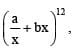the coefficient of x−10 will be

Detailed Solution for Test: Binomial Theorem - 6 - Question 15

Given expansion is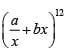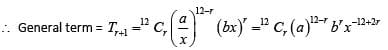Since, we have to find coefficient of x−10
∴ −12 + 2r = −10 ⇒ r = 1
Now, then coefficient of x−10 is 12C1(a)11(b)1 = 12a b

Test: Binomial Theorem - 6 - Question 16

If (1 + ax)n = 1 + 8x + 24x2 + ….., then the values of a and n are equal to

Detailed Solution for Test: Binomial Theorem - 6 - Question 16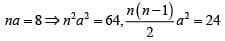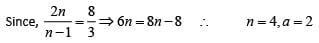Test: Binomial Theorem - 6 - Question 17

The product of middle terms in the expansion of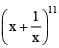is equal to

Detailed Solution for Test: Binomial Theorem - 6 - Question 17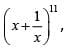it has 12 terms in it’s expansion ,
so there are two middle terms (6th and 7th);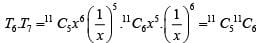Test: Binomial Theorem - 6 - Question 18

The middle term in the expansion of (1 – 2x + x2)n is

Detailed Solution for Test: Binomial Theorem - 6 - Question 18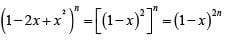Here 2n is even integer, therefore,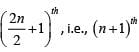term will be the middle term.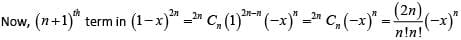Test: Binomial Theorem - 6 - Question 19

The sum of the  binomial coefficients in the expansion of (x−3/4 + ax5/4)n lies between 200 and 400 and the term independent of x equals 448. The value of a is

Detailed Solution for Test: Binomial Theorem - 6 - Question 19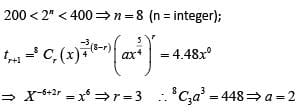Test: Binomial Theorem - 6 - Question 20

23C0 + 23C2+ 23C4 + .. + 23C22 equals

Detailed Solution for Test: Binomial Theorem - 6 - Question 20

Given sum = sum of odd terms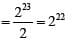## Topic-wise Tests & Solved Examples for IIT JAM Mathematics

27 docs|150 tests
Information about Test: Binomial Theorem - 6 Page
In this test you can find the Exam questions for Test: Binomial Theorem - 6 solved & explained in the simplest way possible. Besides giving Questions and answers for Test: Binomial Theorem - 6, EduRev gives you an ample number of Online tests for practice

## Topic-wise Tests & Solved Examples for IIT JAM Mathematics

27 docs|150 tests(Scan QR code)